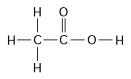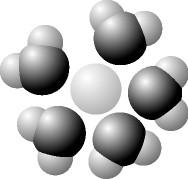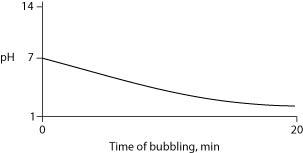# Campbell Biology: Biology Final Flashcards

Set Details Share
created 9 years ago by ashleedavisss
20,455 views
Subjects:
biology, science, life sciences
Page to share:
Embed this setcancel
COPY
code changes based on your size selection
Size:
X

1How many grams of the compound in the figure above would be required to make 1 L of a 0.5 M solution?
(carbon = 12, oxygen = 16, hydrogen = 1)

150

2

A given solution contains 0.0001(10-4) moles of hydrogen ions [H+] per liter. Which of the following best describes this solution?

Acidic: will give h+ to weak acids, but accept h+ from strong acids

3

Which of the following is a hydrophobic material?

wax

4

Many mammals control their body temperature by sweating. Which property of water is most directly responsible for the ability of sweat to lower body temperature?

the absorption of heat by the breaking of hydrogen bonds

5

A 0.01 M solution of a substance has a pH of 2. What can you conclude about this substance?

it is a strong acid that ionizes completely in water

6

A solution contains 0.0000001(10-7) moles of hydroxyl ions [OH-] per liter. Which of the following best describes this solution?

neutral

7

The molecular weight of water is 18 daltons. What is the molarity of 1 liter of pure water? (Hint: What is the mass of 1 liter of pure water?)

55.6 M

8

Which of the following takes place as an ice cube cools a drink?

kinetic energy in the drink decreases

9

Research indicates that acid precipitation can damage living organisms by

washing away certain mineral ions that help buffer soil solution and are essential nutrients for plant growth

10

One idea to mitigate the effects of burning fossil fuels on atmospheric CO2 concentrations is to pipe liquid CO2 into the ocean at depths of 2,500 feet or greater. At the high pressures at such depths, CO2 is heavier than water. What potential effects might result from implementing such a scheme?

Both increased acidity of the deep waters and changes in the growth of bottom dwelling organisms with calcium carbonate shells

11

The slight negative charge at one end of one water molecule is attracted to the slight positive charge of another water molecule. What is this attraction called?

a hydrogen bond

12

When an ionic compound such as sodium chloride (NaCl) is placed in water, the component atoms of the NaCl crystal dissociate into individual sodium ions (Na+) and chloride ions (Cl-). In contrast, the atoms of covalently bonded molecules (e.g., glucose, sucrose, glycerol) do not generally dissociate when placed in aqueous solution. Which of the following solutions would be expected to contain the greatest number of solute particles (molecules or ions)?

1 L of 1.0 M NaCl

13

The bonds that are broken when water vaporizes are

hydrogen bonds between water molecules

14Based on your knowledge of the polarity of water molecules, the solute molecule depicted here is most likely

positively charged

15

You have a freshly prepared 1 M solution of glucose in water. You carefully pour out a 100 mL sample of that solution. How many glucose molecules are included in that 100 mL sample?

6.02 x 1022

16

A slice of pizza has 500 kcal. If we could burn the pizza and use all the heat to warm a 50-L container of cold water, what would be the approximate increase in the temperature of the water? (Note: A liter of cold water weighs about 1 kg.)

10c

17How many grams would be equal to 1 mol of the compound shown in the figure above?
(carbon = 12, oxygen = 16, hydrogen = 1)

60

18

Consider two solutions: solution X has a pH of 4; solution Y has a pH of 7. From this information, we can reasonably conclude that

the concentration of hydrogen ions in solution x is 1,000 times as great as the concentration of hydrogen ions in solution y

19

How many grams of acetic acid (C2H4O2) would you use to make 10 L of a 0.1 M aqueous solution of acetic acid? (Note: The atomic masses, in daltons, are approximately 12 for carbon, 1 for hydrogen, and 16 for oxygen.)

60g

20

Buffers are substances that help resist shifts in pH by

both donating H+ to a solution when bases are added, and accepting H+ when acids are added

21

One mole (mol) of glucose (molecular mass = 180 daltons) is

both 180 grams of glucose and 6.02 x 1023 molecules of glucose

22

Why does evaporation of water from a surface cause cooling of the surface?

the water molecules with the most energy evaporate more readily

23

What is the hydrogen ion [H+] concentration of a solution of pH 8?

10-8 M

24

If the cytoplasm of a cell is at pH 7, and the mitochondrial matrix is at pH 8, this means that

the concentration of H+ ions is tenfold higher in the cytoplasm than in the mitochondrial matrix.

25

Carbon dioxide (CO2) is readily soluble in water, according to the equation CO2 + H2O H2CO3. Carbonic acid (H2CO3) is a weak acid. If CO2 is bubbled into a beaker containing pure, freshly distilled water, which of the following graphs correctly describes the results?26

Hydrophobic substances such as vegetable oil are

nonpolar substances that repel water molecules

27

A beaker contains 100 mL of NaOH solution at pH = 13. A technician carefully pours into the beaker 10 mL of HCl at pH = 1. Which of the following statements correctly describes the results of this mixing?

The pH of the beaker's contents falls

28

In a single molecule of water, two hydrogen atoms are bonded to a single oxygen atom by

polar covalent bonds.

29

The partial negative charge in a molecule of water occurs because

the electrons shared between the oxygen and hydrogen atoms spend more time around the oxygen atom nucleus than around the hydrogen atom nucleus

30

A strong acid like HCl

ionizes completely in an aqueous solution.

31

How many molecules of glucose (C6H12O6 molecular mass = 180 daltons) would be present in 90 grams of glucose?

(90/180) x 6.02 x 1023